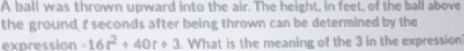### ¿Todavía tienes preguntas de matemáticas?

Pregunte a nuestros tutores expertos
Algebra
PreguntaA ball was thrown upward into the air. The height, in feet, of the ball above the ground. $$f$$ seconds after being thrown can be determined by the expression $$- 16 t ^ { 2} + 40 t + 3$$ . What is the meaning of the $$3$$ in the expression?